Succeed with maths – Part 1

Start this free course now. Just create an account and sign in. Enrol and complete the course for a free statement of participation or digital badge if available.

1 Getting started: puzzles and real-world maths

You probably use maths every day without realising, from working out how much change you might receive, to how long a journey might take to solving puzzles. You’re going to start by looking at some Sudoku puzzles.

In 2005, a puzzle craze known as Sudoku swept across the world, starting in newspapers. These puzzles are closely linked to a branch of mathematics known as graph theory. Sudoku involves putting numbers on a square grid, and its creators claimed that solving it needed just a logical mind – no mathematics required. Most Sudoku puzzles are nine squares long and nine squares wide, a nine-by-nine (9×9) layout, but you are going to look at a smaller example.

You may be wondering what Sudoku has to do with solving real problems. First of all, it will introduce you to working logically, and that is an important general technique that is used often in mathematics. Second, Sudoku puzzles are quite similar to Latin squares, which are used extensively in designing experiments such as finding out how different crops grow with different fertiliser treatments. ‘Latin squares’ are square grids of numbers in which each number occurs just once in every row and column – exactly the same as a Sudoku puzzle!

This happens quite often in mathematics. Very abstract mathematical topics, which seem to have no practical use whatsoever when they are discovered, later turn out to be extremely important in science or technology. Just because it is difficult to see a use at the time does not mean that there will not be some important practical development later!

Activity 1 Do you do Sudoku?

Timing: Allow approximately 10 minutes

Our large square is composed of four blocks, each of which has four smaller squares within. The blocks are framed by thicker lines. There are also four rows across and four columns down, so this setup is a four-by-four (4×4) puzzle. Draw this puzzle for yourself now.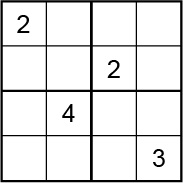Figure 1 Sudoku puzzle

The idea is to arrange the numbers so that each block, row and column contains only one of the numbers 1, 2, 3, and 4. For example, the bottom left-hand block of 4 already has a 4 in it, so you’ll need to put 1, 2 and 3 in the remaining cells in that block. Make sure though that you do not end up with two numbers that are the same in any row or in any column. Try it now! Write your ideas directly on the copy of the diagram you drew. When you have either solved the puzzle or spent about ten minutes on it, read on. Below are some hints and tips if you feel you need more guidance. Click on ‘Reveal comment’ to read these.

Comment

If you have never seen these puzzles before, you may find this quite tricky. There are many different ways that you can tackle this problem. The first step is to try to sort out exactly what you are being asked to do and to make sure you understand the problem.

The next tactic to try is to make the problem simpler by breaking it down into steps and concentrating on just one kind of number. You can choose the number that occurs most often and therefore is the number that you have most information about – in this case, 2.

Now, you need to find the number that makes most sense to fill in that does not violate any of the rules given. Remember: each number (1, 2, 3 and 4) appears only once in each block, row and column. Keep the time limit of ten minutes in mind for the active part of this puzzle. You can spend twice this time if you wish, but don’t go beyond that.

Try a solution now. If you need more guidance, review the next comment.

Comment

In this case, there are two 2s on the grid already, so only two more are needed, one in each of the bottom blocks. There is already a 2 in the first column, so no more 2s can go in that column. The only place for the 2 in the bottom left-hand block is in the square under the 4.

If you find this difficult to follow, draw a pencil line through the column and row that the given 2 is in to show that the 2s in the other blocks cannot be placed in this row or column.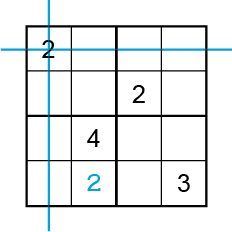Figure 2 Sudoku puzzle with a number added

Similarly, there is already a 2 in the third column, so no more 2s can go in the third column. So the 2 in the bottom right-hand block must go above the 3.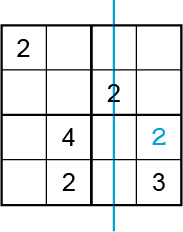Figure 3 Sudoku puzzle with two numbers added

Now, let’s move on. You can see that the bottom row already has a 3 in it, so the 3 in the bottom left-hand block must go next to the 4.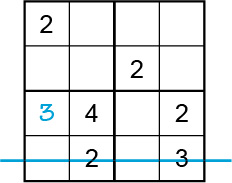Figure 4 Sudoku puzzle with four numbers added

Now look at the third row. Which number is missing?

As more numbers are added, the puzzle gets easier. If you have not finished the puzzle for yourself, try to do so now.

Your final grid should look like this: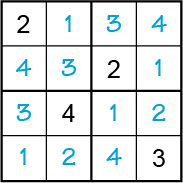Figure 5 Completed Sudoku puzzle

Although there is only one correct answer to each Sudoku puzzle, the approach described in the comment is not the only way to get started on this puzzle. If, for example, you focus on the number 4 in your first move, then you can reason that the block in the lower right-hand corner must have a 4 placed beside the given 3. This would lead to a different start as well as different steps to reason through, but it leads to the same answer.

As there is only one possible arrangement of the numbers in the answer, if you don’t reason your way through, reaching the correct answer is almost impossible.

As you can complete Sudoku with reason alone, you don’t need to carry out any sums to find the solution. This means that there is no need for calculations in your head or on paper. For some of us that might be a bonus! But this might not always be the case and although it is a good idea to have some mental maths skills, there is nothing wrong with using a calculator if you need to. So, before you move onto a maths puzzle that involves doing sums, you’re going to take a quick look at using a calculator.